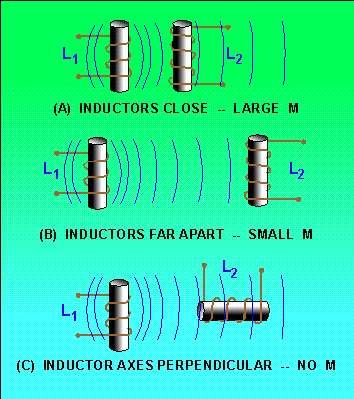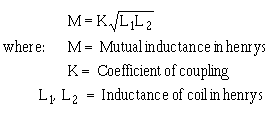Custom SearchWhat is the minimum number of time constants required for the current in an LR circuit to increase to its maximum value? A circuit containing only an inductor and a resistor has a maximum of 12 amperes of applied current flowing in it. After 5 L/R time constants the circuit is opened. How many time constants is required for the current to decay to 1.625 amperes? POWER LOSS IN AN INDUCTOR Since an inductor (coil) consists of a number of turns of wire, and since all wire has some resistance, every inductor has a certain amount of resistance. Normally this resistance is small. It is usually neglected in solving various types of ac circuit problems because the reactance of the inductor (the opposition to alternating current, which will be discussed later) is so much greater than the resistance that the resistance has a negligible effect on the current. However, since some inductors are designed to carry relatively large amounts of current, considerable power can be dissipated in the inductor even though the amount of resistance in the inductor is small. This power is wasted power and is called COPPER LOSS. The copper loss of an inductor can be calculated by multiplying the square of the current in the inductor by the resistance of the winding (I2R). In addition to copper loss, an iron-core coil (inductor) has two iron losses. These are called HYSTERESIS LOSS and EDDY-CURRENT LOSS. Hysteresis loss is due to power that is consumed in reversing the magnetic field of the inductor core each time the direction of current in the inductor changes. Eddy-current loss is due to heating of the core by circulating currents that are induced in the iron core by the magnetic field around the turns of the coil. These currents are called eddy currents and circulate within the iron core only. All these losses dissipate power in the form of heat. Since this power cannot be returned to the electrical circuit, it is lost power. Q.11 State three types of power loss in an inductor. MUTUAL INDUCTANCE Whenever two coils are located so that the flux from one coil links with the turns of the other coil, a change of flux in one coil causes an emf to be induced in the other coil. This allows the energy from one coil to be transferred or coupled to the other coil. The two coils are said to be coupled or linked by the property of MUTUAL INDUCTANCE (M). The amount of mutual inductance depends on the relative positions of the two coils. This is shown in figure 2-12. If the coils are separated a considerable distance, the amount of flux common to both coils is small and the mutual inductance is low. Conversely, if the coils are close together so that nearly all the flux of one coil links the turns of the other, the mutual inductance is high. The mutual inductance can be increased greatly by mounting the coils on a common iron core. Figure 2-12. - The effect of position of coils on mutual inductance (M).Two coils are placed close together as shown in figure 2-13. Coil 1 is connected to a battery through switch S, and coil 2 is connected to an ammeter (A). When switch S is closed as in figure 2-13(A), the current that flows in coil 1 sets up a magnetic field that links with coil 2, causing an induced voltage in coil 2 and a momentary deflection of the ammeter. When the current in coil 1 reaches a steady value, the ammeter returns to zero. If switch S is now opened as in figure 2-13(B), the ammeter (A) deflects momentarily in the opposite direction, indicating a momentary flow of current in the opposite direction in coil 2. This current in coil 2 is produced by the collapsing magnetic field of coil 1. Figure 2-13. - Mutual inductance.Q.12 Define mutual inductance. FACTORS AFFECTING MUTUAL INDUCTANCE The mutual inductance of two adjacent coils is dependent upon the physical dimensions of the two coils, the number of turns in each coil, the distance between the two coils, the relative positions of the axes of the two coils, and the permeability of the cores. The COEFFICIENT OF COUPLING between two coils is equal to the ratio of the flux cutting one coil to the flux originated in the other coil. If the two coils are so positioned with respect to each other so that all of the flux of one coil cuts all of the turns of the other, the coils are said to have a unity coefficient of coupling. It is never exactly equal to unity (1), but it approaches this value in certain types of coupling devices. If all of the flux produced by one coil cuts only half the turns of the other coil, the coefficient of coupling is 0.5. The coefficient of coupling is designated by the letter K. The mutual inductance between two coils, L1 and L2, is expressed in terms of the inductance of each coil and the coefficient of coupling K. As a formula:One 10-H coil and one 20-H coil are connected in series and are physically close enough to each other so that their coefficient of coupling is 0.5. What is the mutual inductance between the coils?Q.13 When are two circuits said to be coupled? Q.14 What is meant by the coefficient of coupling?Integrated Publishing, Inc. - A (SDVOSB) Service Disabled Veteran Owned Small Business